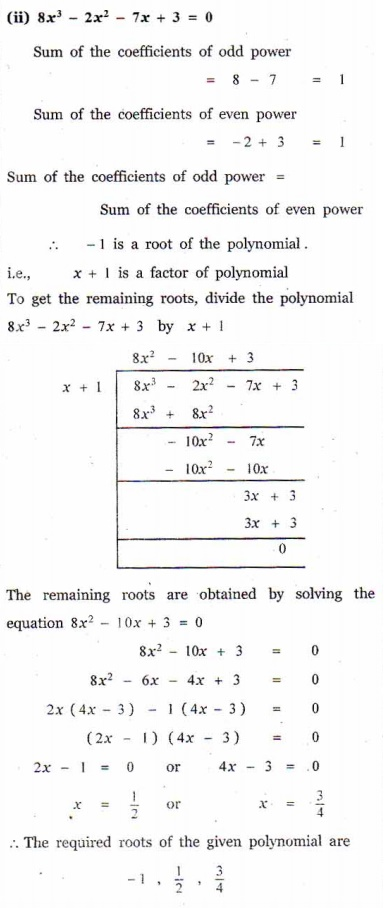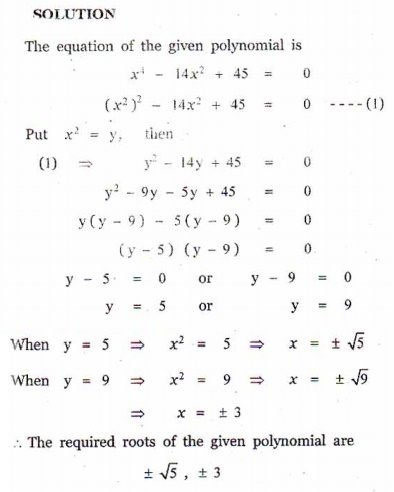Home | | Maths 12th Std | Exercise 3.3: Polynomials with Additional Information

# Exercise 3.3: Polynomials with Additional Information

Maths Book back answers and solution for Exercise questions - Mathematics : Theory of Equations: Polynomials with Additional Information: Exercise problems with Questions, Answers, Solution, Explanation

EXERCISE 3.3

1. Solve the cubic equation : 2x3 - x2 -18+ 9 = 0 if sum of two of its roots vanishes.2. Solve the equation 9x3 - 36x2 + 44-16 = 0 if the roots form an arithmetic progression.3. Solve the equation 3x3 - 26x2 + 52- 24 = 0 if its roots form a geometric progression.4. Determine and solve the equation 2x3 - 6x2 + 3= 0 if one of its roots is twice the sum of the other two roots.5. Find all zeros of the polynomial x6 - 3x5 - 5x4 + 22x3 - 39x2 - 39+135 , if it is known that1+ 2and √3 are two of its zeros.6. Solve the cubic equations : (i) 2x3 - 9x2 +10= 3 , (ii) 8x3 - 2x2 - 7+ 3 = 0.7. Solve the equation : x4 -14x2 + 45 = 0.